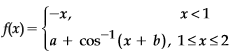#### If the functionis differentiable at x = 1, thenis equal  to : Option 1)Option 2)Option 3)Option 4)As we learnt in

Rule for continuous -

A function is continuous at  x = a if and only ifL.H.L    R.H.L   value at  x = a.

- wherein

Where1+b=0        b=-1

Now f(x) will be continuous at x=1Option 1)This option is incorrect.

Option 2)This option is incorrect.

Option 3)This option is correct.

Option 4)This option is incorrect.

#### divya.saini